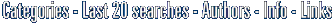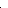GraphNow

Country: China - City: Chongqing
Web Site: Web site

GraphNow products:Equation grapher is an easy-to-use software for 2D function graphing.Equation grapher is an easy-to-use software for 2D function graphing.Equation grapher is an easy-to-use software for 2D function graphing.
See also:grapher software, function software, equation software, graph software, graphs software, table software,Math tool for high school math, middle school math teaching and studying. Function graphing and analyzing, sequence of number, analytic geometry and solid geometry.Visual Complex is a graph software to create graph of complex function. 3D function graphs and 2D color maps can be created with this grapher.Math calculator, also derivative calculator, integral calculator, calculus calculator, expression calculator, equation solver, can be used to calculate expression, derivative, root, extremum, integral.Scientific data visualization software. Creating vertex, grid and surface model, color map, contour plot, vector plot, 4D scatter, 4D slice and 4D vector.Function Grapher is a easy-to-use software for 2D, 2.5D and 3D function graphing and animation.
See also:grapher software, function software, equation software, graph software, graphs software, table software,Visual Calculus is an easy-to-use calculus grapher for Graphing limit, derivative function, integral, 3D vector, series, ode etc. Which can create 2D, 2.5D and 3D function graphs, animations and table graphs.CategoriesKeep yourself updated, subscribe to the soft14 Newsletter now, its free! Enter your email here: